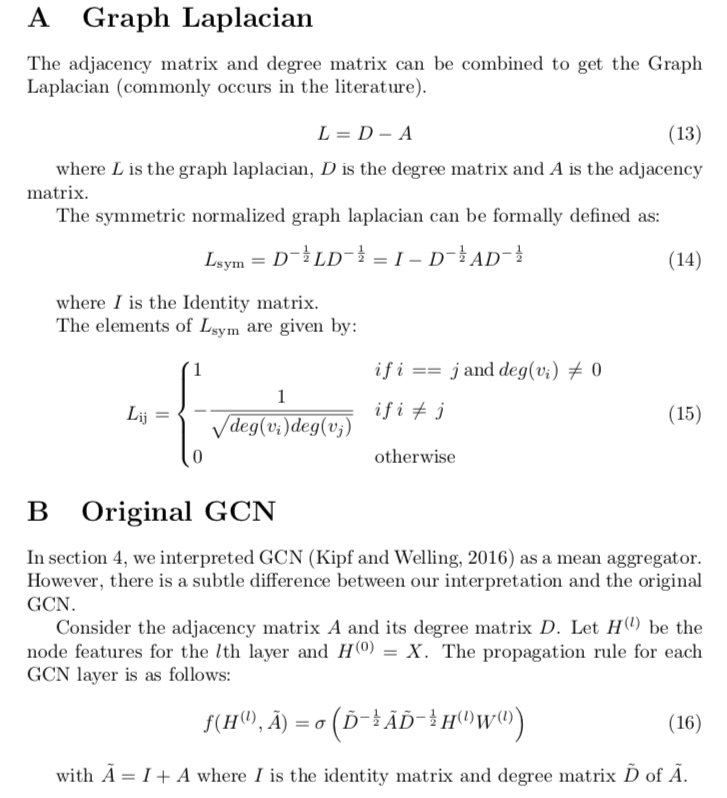# Graph Neural Networks图神经网络（一）

Posted by JoselynZhao on April 12, 2020

Author: Nihai V. Nayak (March 2020)

@toc

# 01 Introduction

• Graph Neural Networks (GNN) is a type of neural network which learns the structure of a graph.
• Learning graph structure allows us to represent the nodes of the graph in the euclidean space which can be useful for several downstream machine learning tasks.
• Recent work on GNN has shown impressive performance on link prediction（链接预测）, graph classification（图分类）and semi-supervised tasks (Hamil- ton et al., 2017b).

# 02 Basics

• Graphs consist of a set of nodes V and edges E.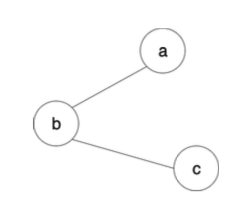fig.1
• They are normally represented with an unweighted adjacency matrix(邻接矩阵) A, which consists of 1s and 0s.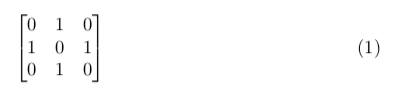• The degree matrix(度矩阵) is a diagonal matrix （对角矩阵）that contains information about the degree of each node.

不区分出度和入度

• the degree matrix for fig. 1 is as follows: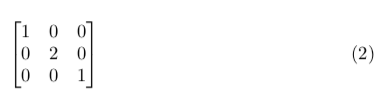• from Figure 1 the neighbours of the node b are: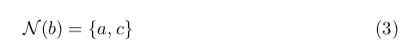where N(.) is the neighbour function. Likewise, the neighbor of the node a is: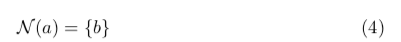• Therefore, we need a neural network that can deal with the varying number of neighbors.

# 03 Learning on Graphs

A basic GNN layer consists of two steps：

• AGGREGATE：For a given node, ==the AGGREGATE step applies a permutation invariant function to its neighbors to generate the aggregated（汇总的） node feature==
• COMBINE: For a given node, ==COMBINE step passes the aggregated node feature to a learnable layer to generate node embedding for the GNN layer==

The GNN layers are stacked together（堆叠在一起） to increase the receptive field of clustering. In Fig. 2 we have 2 GNN layers. This means that for every node, we aggregate over the 2-hop neighborhood.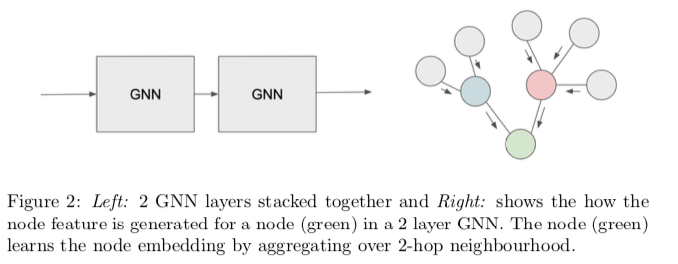AGGREGATE and COMBINE steps are sometimes called message passing phase and readout phase （读出）in the message passing neural network framework (Gilmer et al., 2017).

## 03.1 Formal Definition

• Consider the Graph G = (V,E), where V is the set of node with features Xv. There are two main steps - AGGREGATE and COMBINE at each l-th layer of the GNN: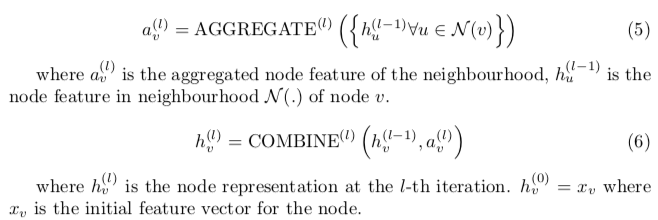# 04 Graph Convolutional Networks （GCN）

• GCN (Kipf and Welling, 2016) is a graph neural network technique that makes use of the symmetrically normalized graph laplacian（对称归一化图拉普拉斯算子） to compute the node embeddings.
• Each GCN block receives node features from the (l−1)th GCN block, i.e. the node features from the previous layer is passed to the next GCN layer, which allows for greater laplacian smoothing（拉普拉斯平滑） (Li et al., 2018).

## 04.1 Aggregate

Since we don’t always have access to the entire graph structure to compute the symmetrically normalized graph laplacian, GCN Aggregator has been interpreted as a Mean Aggregator（均值聚合器） (Xu et al., 2018a). Therefore,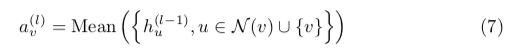For each node v, the algorithm takes the mean of the neighborhood node features along with its feature (self-loop) to compute the aggregated node feature for the l-th layer. This formulation makes GCN an inductive graph neural network（归纳图神经网络）.

## 04.2 Combine

The aggregated node feature av is multiplied with a learnable weight matrix followed by a non-linear activation (ReLU) to get the node feature hv for the lth layer,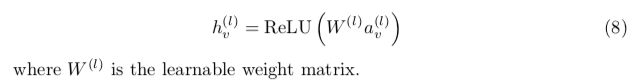# 05 GraphSage

• Graphsage (Hamilton et al., 2017a) is a spatial graph neural network algorithm （空间图神经网络算法）that learns node embeddings by sampling and aggregating neighbors from multiple hops.
• Graphsage, unlike GCN, is an inductive learning algorithm（归纳学习算法） which means that ==it does not require the entire graph structure during learning==.
• In the original Graphsage paper, the authors describe multiple aggregators-Mean, Pooling and LSTM Aggregator.
• In this section, we’ll be describing the Pool Aggregator and the combine from the original paper.
• For simplicity, ==we do not sample the neighborhood nodes as well.==

## 05.1 Aggregate

• Graphsage makes use of a max-pooling aggregator to compute an aggregated node feature.
• All the neighborhood node features are passed through a linear layer.
• We then pass these features through a non-linear activation before applying max filter. Formally,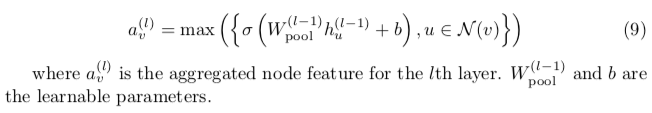## 05.2 Combine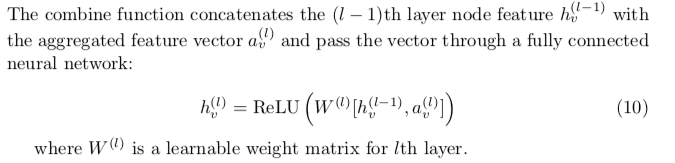# 06 Graph Attention Network（GAT）

• Graph Attention Network (Veliˇckovi ́c et al., 2018) is a spatial graph neural network technique that uses ==Self Attention to aggregate the neighborhood node features.==
• Self Attention is equivalent to computing a weighted mean of the neighbourhood node features.
• The original paper uses K masked self attention modules to aggregate node features. For simplicity, we consider only one self attention block.

## 06.1 Aggregate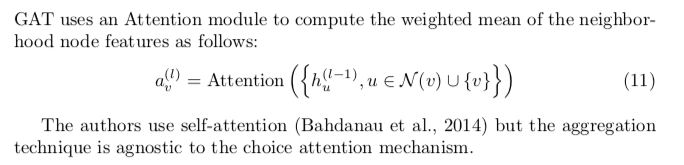## 06.2 Combine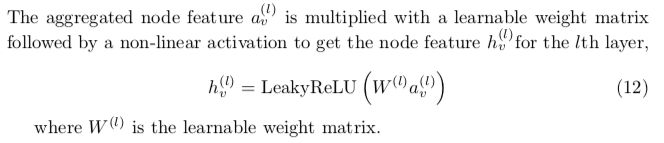# 07 Related Works

• Apart from GCN, Graphsage, and GAT, there are few more works that frequently appear in the literature.
• SGN (Wu et al., 2019) simplifies GCN by removing non-linearity between the GCN layers. Furthermore, to achieve the same “receptive field”（感受野） of having K GCN layers, they take the graph laplacian to the power of K and show equivalent performance.
• Xu et al. (2018b) introduced jumping knowledge networks that aggregate node features generated from all the previous layers to compute the final vector using mean or max-pooling. This allows the model to learn better structure-aware representations（结构感知表示） from different neighborhood aggregations. The works described so far have not taken advantage of the relations in the graph.
• R-GCN (Schlichtkrull et al., 2018) and Directed-GCN (Marcheggiani and Titov, 2017) extend GCN to relational graphs.
• Bahdanau, D., Cho, K., and Bengio, Y. (2014). Neural machine translation by jointly learning to align and translate. arXiv preprint arXiv:1409.0473.
• Gilmer, J., Schoenholz, S. S., Riley, P. F., Vinyals, O., and Dahl, G. E. (2017). Neural message passing for quantum chemistry. In Proceedings of the 34th International Conference on Machine Learning-Volume 70, pages 1263–1272. JMLR. org.
• Hamilton, W., Ying, Z., and Leskovec, J. (2017a). Inductive representation learning on large graphs. In Advances in Neural Information Processing Sys- tems, pages 1024–1034.
• Hamilton, W. L., Ying, R., and Leskovec, J. (2017b). Representation learning on graphs: Methods and applications. IEEE Data Eng. Bull., 40:52–74.
• Kipf, T. N. and Welling, M. (2016). Semi-supervised classification with graph convolutional networks.
• Li, Q., Han, Z., and Wu, X.-M. (2018). Deeper insights into graph convolutional networks for semi-supervised learning. In Thirty-Second AAAI Conference on Artificial Intelligence.
• Marcheggiani, D. and Titov, I. (2017). Encoding sentences with graph convolu- tional networks for semantic role labeling. arXiv preprint arXiv:1703.04826.
• Schlichtkrull, M., Kipf, T. N., Bloem, P., Van Den Berg, R., Titov, I., and Welling, M. (2018). Modeling relational data with graph convolutional net- works. In European Semantic Web Conference, pages 593–607.
• Springer. Veliˇckovi ́c, P., Cucurull, G., Casanova, A., Romero, A., Li`o, P., and Bengio, Y. (2018). Graph Attention Networks. International Conference on Learning Representations.
• Wu, F., Souza, A., Zhang, T., Fifty, C., Yu, T., and Weinberger, K. (2019). Simplifying graph convolutional networks. In International Conference on Machine Learning, pages 6861–6871.
• Xu, K., Hu, W., Leskovec, J., and Jegelka, S. (2018a). How powerful are graph neural networks?
• Xu, K., Li, C., Tian, Y., Sonobe, T., Kawarabayashi, K.-i., and Jegelka, S. (2018b). Representation learning on graphs with jumping knowledge net- works. arXiv preprint arXiv:1806.03536.